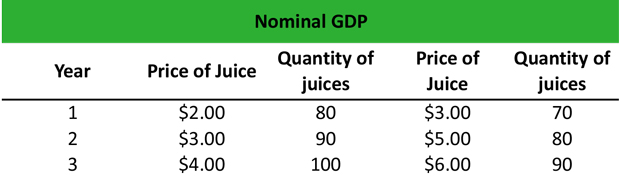# What is Nominal GDP?

Definition: Nominal GDP, or gross domestic product, measures the value of all finished goods and services produced by a country at their current market prices. Typically, economists use a gross domestic deflator to convert nominal GDP to real GDP.

## What Does Nominal GDP Mean?

What is the definition of nominal GDP? Nominal GDP includes all the changes in the prices of finished goods and services that took place in one year due to inflation or deflation.

It can be measured by using production, expenditure or income. The GDP deflator is a conversion factor that adjusts nominal to real gross domestic product by measuring the level of current prices compared to the level of prices of the base year. The GDP deflator in the base year is always equal to 100.

Let’s look at an example.

## Example

Anna is an economist working at the Bureau of Labor Statistics. She wants to calculate the difference between nominal GDP and real GDP for a particular good in the economy. Anna creates a table using Year 1 as a base year, and she inputs the data she collected from her agency.Anna calculates the nominal GDP formula by multiplying the price x quantity of each year:

Year 1: (\$2 x 80) + (\$3 x 70) = \$160 + \$210 = \$370
Year 2: (\$3 x 90) + (\$5 x 80) = \$270 + \$400 = \$670
Year 3: (\$4 x 100) + (\$6 x 90) = \$400 + \$540 = \$940

Then, she calculates the real GPD equation by multiplying the price of the base year 1 x quantity of each year:

Year 1: (\$2 x 80) + (\$3 x 70) = \$160 + \$210 = \$370
Year 2 (\$2 x 90) + (\$3 x 80) = \$180 + \$240 = \$420
Year 3: (\$2 x 100) + (\$3 x 90) = \$200 + \$270 = \$470

Finally, Anna uses the GDP to adjust the nominal GDP to real GDP as follows:

GDP Deflator = Nominal GDP / Real GDP x 100

Year 1: GDP deflator = \$370 / \$370 x 100 = 100
Year 2: GDP deflator = \$670 / \$420 x 100 = \$160
Year 3: GDP deflator = \$940 / \$470 x 100 = \$200

## Summary Definition

Define Nominal GDP: Nominal gross domestic product is an economic formula that measures the total market value of all goods and services produced by a country in a single year.